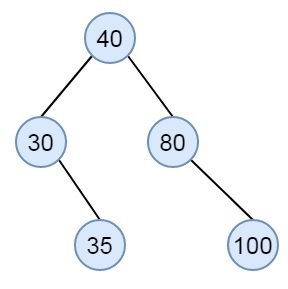# Check if a given array can represent Preorder Traversal of Binary Search Tree in C++

Suppose we have a list of elements in an array, we have to check, whether the elements can be the preorder traversal of a binary search tree or not. Suppose a sequence is like {40, 30, 35, 80, 100}, then the tree will be like −We can solve this using a stack. We have to follow these steps to solve this problem.

• Define empty stack
• set root as negative infinity
• for every element in preorder sequence, do the following −
• if the element is smaller than current root, return false
• Keep removing elements from stack while the element is greater than stack top, and make the lastly removed element as root.
• push the element into stack

## Example

#include <iostream>
#include <stack>
using namespace std;
bool isValidPreorder(int pre[], int n) {
stack<int> stk;
int root = INT_MIN;
for (int i=0; i<n; i++) {
if (pre[i] < root)
return false;
while (!stk.empty() && stk.top()<pre[i]) {
root = stk.top();
stk.pop();
}
stk.push(pre[i]);
}
return true;
}
int main() {
int pre[] = {40, 30, 35, 80, 100};
int n = sizeof(pre)/sizeof(pre);
if(isValidPreorder(pre, n))
cout << "This can form BST";
else
cout << "This can not form BST";
}

## Output

This can form BST